No User logged in, System Language:
sound waves and measurements
As before mentioned, in this class we will examine some of the main qualities of a waveform. The relations between waveforms and their behaviour are ruled by precise mathematical and trigonometrical formulas, which makes it possible to predict and calculate most aspects of sound.

First of all let's start by saying that a sine waveform does not actually exists in nature. Just as the linear motion, a sine is a physical model that is easier to study and serves the purpose of representing a phenomenon otherwise too complex to describe. Many variables like inertia, friction, mass of the air particles and the vibrating objects make such a regular motion simply impossible: we can get very close to it, thanks to some devices called oscillators which generate a very regular waveform, but we cannot get any better than this.

Bearing this in mind, we will now take a closer look to our wave: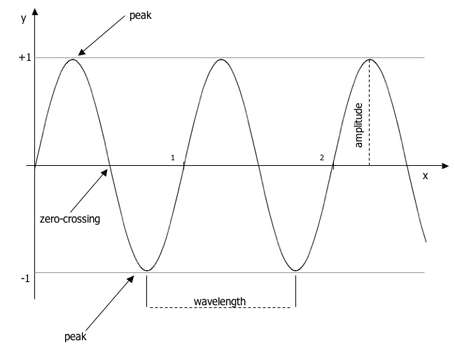The values on the X axis are time in seconds and the values of 1 and -1 on the Y axis the maximum compression and rarefaction respectively; these values define the peaks of the wave, while the intersections between the waveform and the X axis are called zero-crossing points. The distance between two peaks is called wavelength, while the distance between a zero-crossing and a peak is called amplitude. These values are very important to identify sound characteristics: the amplitude generally determines how loud the sound is perceived, while the wavelength (and thus the frequency) determines its pitch.

The above example describes the movement of a particle from its rest position, to it maximum compression, then back to its rest position, then maximum rarefaction, then back to its rest position. This is called a cycle. The time that this whole cycle takes to complete is called period. The amount of cycles that the particle completes in one second is called frequency. Needless to say these variables are strictly interdependent. Even the slightest variation of one of the three, will cause a change in the way we perceive the sound. Before getting into the details of sound perception, we should take a look at how these values depend on each other:

Period (conventionally represented by a capital 'T') is inversely proportional to frequency (represented by an ƒ). Period is a time value and is measured in seconds (s).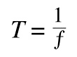So, the higher the frequency, the lower the time it takes to complete a cycle.

And as follows, the frequency is inversely proportional to the period. The frequency is measured in Hertz (Hz), a unit that is specifically employed to measure cyclic events in a unit of time.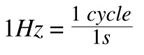Very often a multiple of Hz is used to measure high frequencies, the KiloHertz (KHz).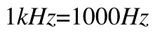The higher the time that it takes to complete a cycle, the lower the frequency.

The wavelength (indicated by a Greek lambda) is also related to the frequency, but there is another variable that comes into place: the speed of sound in the medium, usually indicated by a capital C. The speed of sound in air at 20°C is approximately 344m/s. This speed changes with the air temperature, density, humidity, the wind and many other factors so that it would be extremely complicated to keep track of it continuously. Thus, we tend to treat C as a constant, which leads to a certain degree of approximation of course; Fortunately, this approximation is tolerable in most sound engineering applications.

So, being the relation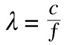We can see that the wavelength is inversely proportional to the frequency, which means that higher frequencies will have a shorter wavelength.

How long can a sound wave be? Of course it depends on its frequency, so let's see how long are the waves of a few sounds:

A very common pure tone, is 1KHz: this is used as a test tone in many machines and in sound engineering in general.

its wavelength will be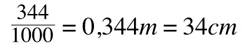as we will discuss more in details later on, a human ear is capable of hearing sounds from a frequency of 20Hz to 20KHz.

Tone: 20Hz this 20Hz pure tone's wavelength is 17 meters

Tone: 20KHz while this 20KHz's will be a tiny 0.0172m or 1.72cm

And if could not hear any of the two sounds, don't worry... most sound equipment is not able to reproduce extremely high or low frequencies (despite what you read on the specs).

so, before continuing let's summarise it all in a table. Make sure you are familiar with all the different symbols and concepts before continuing:

 measurement symbol measured in Frequency ƒ Hz (and KHz) Period T s (seconds) Wavelength lambda m (meters) Speed C m/s (meters per second)

There are another two important measurements which we you must be familiar with:

Amplitude: we mentioned it but did not get into details with it

Phase: it is a little more complicated, because it is a measurement that only takes place when more than one sound wave is involved

Let's take a break before them... We are sure you all fancy a little maths now!